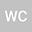Two methods for constructing the effective Laplace value of gravitational bosons
•Wen-Xiang ChenDepartment of Astronomy, School of Physics and Materials Science, GuangZhou University

Corresponding Author:1112019001@e.gzhu.edu.cn

Author Profile## Abstract

This paper introduces two methods for constructing the effective Laplace value of gravitational bosons: the CCWZ method and the topological method, and then uses these two methods to calculate the symmetry breaking SO(4)/SO(3) of the effective Laplace value of the gravitational boson. By comparing the results, it is found that the effective Laplace value constructed by the two methods is consistent, which further proves that the topological method is useful in constructing the effective Laplace value of the gravitational boson effectiveness.